PSA9B2B - Student-Facing Tasks---7.1 Lesson 2: Corresponding Parts and Scale Factors: Student Facing Tasks (7.G.A.1)
Part A)

Find the product mentally.Type your answer below as a number (example: 5, 3.1, 4 1/2, or 3/2):
Part B)Type your answer below as a number (example: 5, 3.1, 4 1/2, or 3/2):
Part C)Type your answer below as a number (example: 5, 3.1, 4 1/2, or 3/2):
Part A)

Here is a figure and two copies, each with some points labeled.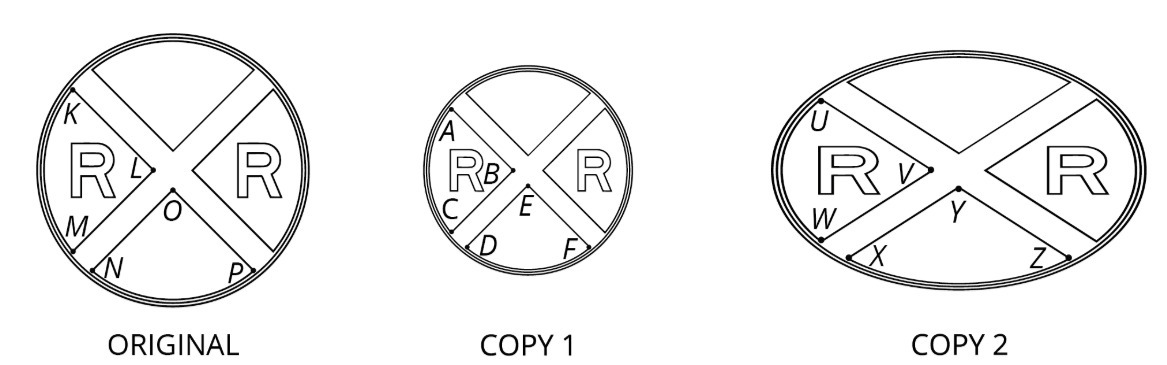Complete this table to show corresponding parts in the three figures.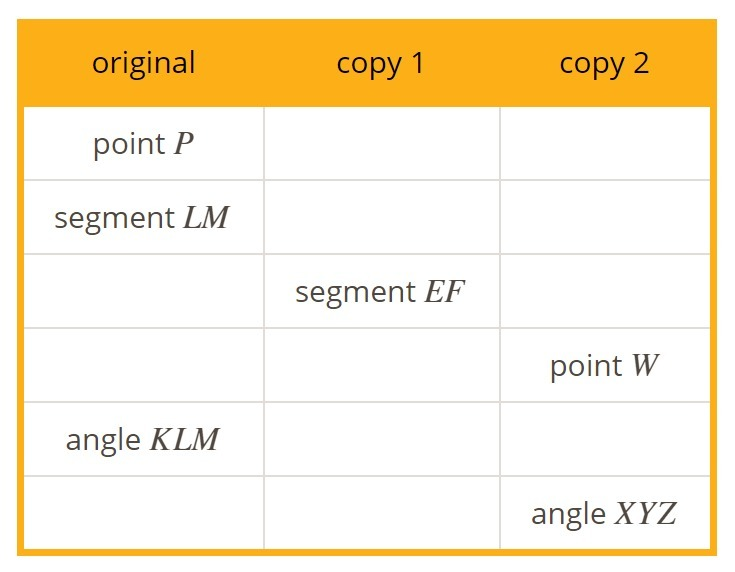Create and fill in the table using the table button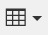Part B)

Is either copy a scaled copy of the original figure?

Select one:
Part C)

Part D)

Use tracing paper to compare angle KLM with its corresponding angles in Copy 1 and Copy 2. What do you notice?

Part E)

Use tracing paper to compare angle NOP with its corresponding angles in Copy 1 and Copy 2. What do you notice?

Part A)

Here is Triangle O, followed by a number of other triangles.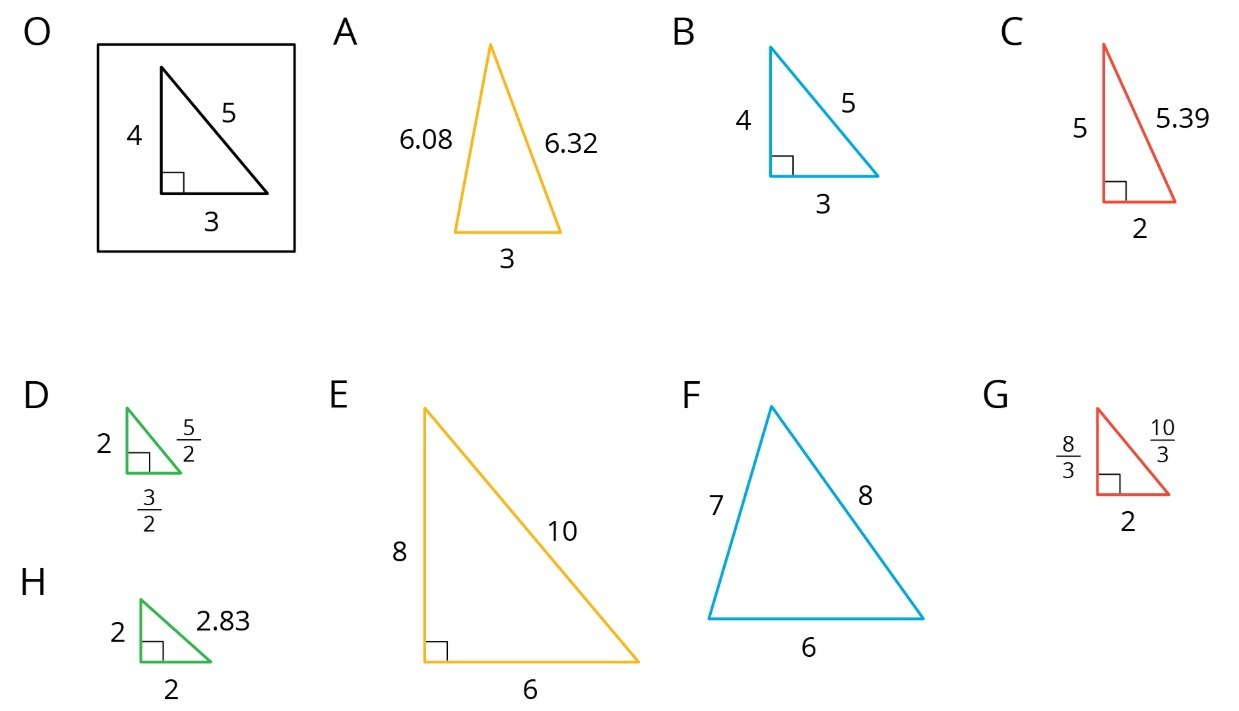Which triangles are scaled copy of Triangle O?

Select all that apply:
Part B)

List all the triangles that are scaled copies in the table. Record the side lengths that correspond to the side lengths of Triangle O listed in each column.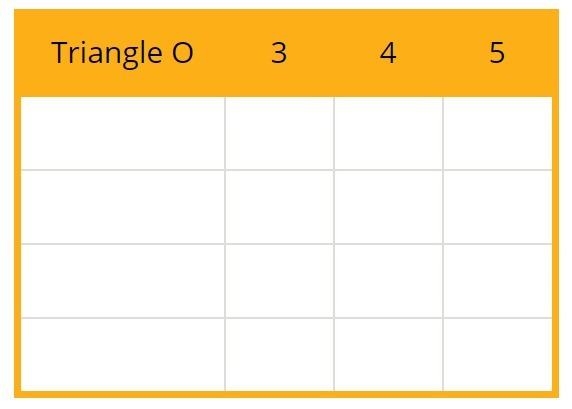Create and fill in the table using the table buttonPart C)

Explain or show how each copy has been scaled from the original (Triangle O).

Part A)

Polygon PQRS is a scaled copy of polygon ABCD.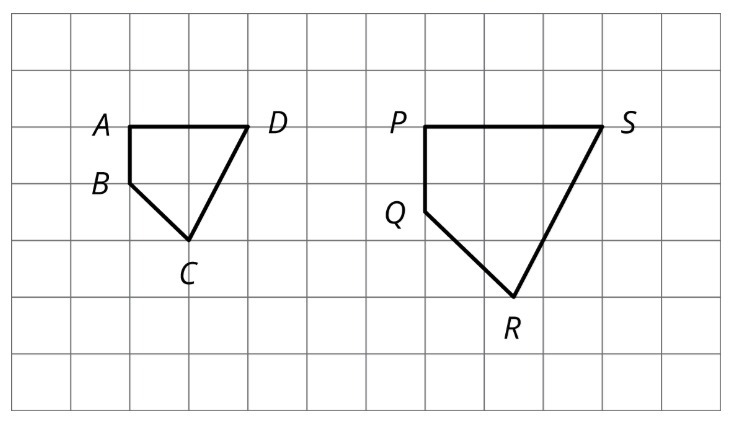Name the angle in the scaled copy that corresponds to angle ABC.

Select one:
Part B)

Name the segment in the scaled copy that corresponds to segment AD.

Select one:
Part C)

What is the scale factor from polygon ABCD to polygon PQRS?

Type your answer below as a number (example: 5, 3.1, 4 1/2, or 3/2):